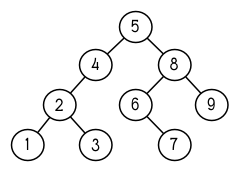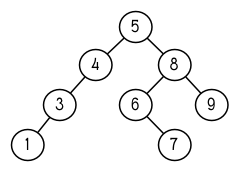# 第四章：特殊資料結構¶

Common Lisp 還有另一種資料結構：實體（instance）。實體將在 11 章討論，講述 CLOS。

## 4.1 陣列 (Array)¶

> (setf arr (make-array '(2 3) :initial-element nil))
#<Simple-Array T (2 3) BFC4FE>


Common Lisp 的陣列至少可以有七個維度，每個維度至多可以有 1023 個元素。

:initial-element 實參是選擇性的。如果有提供這個實參，整個陣列會用這個值作爲初始值。若試著取出未初始化的陣列內的元素，其結果爲未定義（undefined）。

aref 取出陣列內的元素。與 Common Lisp 的存取函數一樣， aref 是零索引的（zero-indexed）：

> (aref arr 0 0)
NIL


> (setf (aref arr 0 0) 'b)
B
> (aref arr 0 0)
B


#2a((b nil nil) (nil nil nil))


> (setf *print-array* t)
T
> arr
#2A((B NIL NIL) (NIL NIL NIL))


> (setf vec (make-array 4 :initial-element nil))
#(NIL NIL NIL NIL)


> (vector "a" 'b 3)
#("a" b 3)


> (svref vec 0)
NIL


svref 內的 “sv” 代表“簡單向量”（“simple vector”），所有的向量預設是簡單向量。 

## 4.3 字元與字串 (Strings and Characters)¶

> (sort "elbow" #'char<)
"below"


> (aref "abc" 1)
#\b


> (char "abc" 1)
#\b


> (let ((str (copy-seq "Merlin")))
(setf (char str 3) #\k)
str)


> (equal "fred" "fred")
T
> (equal "fred" "Fred")
NIL
>(string-equal "fred" "Fred")
T


Common Lisp 提供大量的操控、比較字串的函數。收錄在附錄 D，從 364 頁開始。

> (format nil "~A or ~A" "truth" "dare")
"truth or dare"


> (concatenate 'string "not " "to worry")
"not to worry"


## 4.4 序列 (Sequences)¶

> (mirror? "abba")
T


> (elt '(a b c) 1)
B


(defun mirror? (s)
(let ((len (length s)))
(and (evenp len)
(do ((forward 0 (+ forward 1))
(back (- len 1) (- back 1)))
((or (> forward back)
(not (eql (elt s forward)
(elt s back))))
(> forward back))))))


:key 應用至每個元素的函數 identity
:test 作來比較的函數 eql
:from-end 若爲真，反向工作。 nil
:start 起始位置 0
:end 若有給定，結束位置。 nil

> (position #\a "fantasia")
1
> (position #\a "fantasia" :start 3 :end 5)
4


> (position #\a "fantasia" :from-end t)
7


:key 關鍵字參數是序列中每個元素在被考慮之前，應用至元素上的函數。如果我們說，

> (position 'a '((c d) (a b)) :key #'car)
1


:test 關鍵字參數接受需要兩個實參的函數，並定義了怎樣是一個成功的匹配。預設函數爲 eql 。如果你想要匹配一個列表，你也許想使用 equal 來取代：

> (position '(a b) '((a b) (c d)))
NIL
> (position '(a b) '((a b) (c d)) :test #'equal)
0


:test 關鍵字參數可以是任何接受兩個實參的函數。舉例來說，給定 < ，我們可以詢問第一個使第一個參數比它小的元素位置：

> (position 3 '(1 0 7 5) :test #'<)
2


(defun second-word (str)
(let ((p1 (+ (position #\  str) 1)))
(subseq str p1 (position #\  str :start p1))))


> (second-word "Form follows function")
"follows"


> (position-if #'oddp '(2 3 4 5))
1


position-if 接受除了 :test 之外的所有關鍵字參數。

> (find #\a "cat")
#\a

> (find-if #'characterp "ham")
#\h


(find-if #'(lambda (x)
(eql (car x) 'complete))
lst)


(find 'complete lst :key #'car)


> (remove-duplicates "abracadabra")
"cdbra"


(reduce #'fn '(a b c d))


(fn (fn (fn 'a 'b) 'c) 'd)


> (reduce #'intersection '((b r a d 's) (b a d) (c a t)))
(A)


## 4.5 範例：解析日期 (Example: Parsing Dates)¶

(defun tokens (str test start)
(let ((p1 (position-if test str :start start)))
(if p1
(let ((p2 (position-if #'(lambda (c)
(not (funcall test c)))
str :start p1)))
(cons (subseq str p1 p2)
(if p2
(tokens str test p2)
nil)))
nil)))

(defun constituent (c)
(and (graphic-char-p c)
(not (char= c #\ ))))


> (tokens "ab12 3cde.f" #'alpha-char-p 0)
("ab" "cde" "f")


> (tokens "ab12 3cde.f gh" #'constituent 0)
("ab12" "3cde.f" "gh")


> (parse-date "16 Aug 1980")
(16 8 1980)

(defun parse-date (str)
(let ((toks (tokens str #'constituent 0)))
(list (parse-integer (first toks))
(parse-month (second toks))
(parse-integer (third toks)))))

(defconstant month-names
#("jan" "feb" "mar" "apr" "may" "jun"
"jul" "aug" "sep" "oct" "nov" "dec"))

(defun parse-month (str)
(let ((p (position str month-names
:test #'string-equal)))
(if p
(+ p 1)
nil)))


parse-date 使用 tokens 來解析日期字串，接著呼叫 parse-monthparse-integer 來轉譯年、月、日。要找到月份，呼叫 parse-month ，由於使用的是 string-equal 來匹配月份的名字，所以輸入可以不分大小寫。要找到年和日，呼叫內建的 parse-integerparse-integer 接受一個字串並返回對應的整數。

(defun read-integer (str)
(if (every #'digit-char-p str)
(let ((accum 0))
(dotimes (pos (length str))
(setf accum (+ (* accum 10)
(digit-char-p (char str pos)))))
accum)
nil))


## 4.6 結構 (Structures)¶

(defun block-height (b) (svref b 0))


(defstruct point
x
y)


2.3 節提過， Lisp 程式可以寫出 Lisp 程式。這是目前所見的明顯例子之一。當你呼叫 defstruct 時，它自動生成了其它幾個函數的定義。有了宏以後，你將可以自己來辦到同樣的事情（如果需要的話，你甚至可以自己寫出 defstruct ）。

(setf p (make-point :x 0 :y 0))
#S(POINT X 0 Y 0)


> (point-x p)
0
> (setf (point-y p) 2)
2
> p
#S(POINT X 0 Y 2)


> (point-p p)
T
> (typep p 'point)
T


(defstruct polemic
(type (progn
(format t "What kind of polemic was it? ")
(effect nil))


> (make-polemic)
What kind of polemic was it? scathing
#S(POLEMIC :TYPE SCATHING :EFFECT NIL)


(defstruct (point (:conc-name p)
(:print-function print-point))
(x 0)
(y 0))

(defun print-point (p stream depth)
(format stream "#<~A, ~A>" (px p) (py p)))


:conc-name 關鍵字參數指定了要放在欄位前面的名字，並用這個名字來生成存取函數。預設是 point- ；現在變成只有 p 。不使用預設的方式使程式的可讀性些微降低了，只有在需要常常用到這些存取函數時，你才會想取個短點的名字。

:print-function 是在需要顯示結構出來看時，指定用來打印結構的函數 ── 需要顯示的情況比如，要在頂層顯示時。這個函數需要接受三個實參：要被印出的結構，在哪裡被印出，第三個參數通常可以忽略。  我們會在 7.1 節討論流（stream）。現在來說，只要知道流可以作爲參數傳給 format 就好了。

> (make-point)
#<0,0>


## 4.7 範例：二元搜索樹 (Example: Binary Search Tree)¶(defstruct (node (:print-function
(lambda (n s d)
(format s "#<~A>" (node-elt n)))))
elt (l nil) (r nil))

(defun bst-insert (obj bst <)
(if (null bst)
(make-node :elt obj)
(let ((elt (node-elt bst)))
(if (eql obj elt)
bst
(if (funcall < obj elt)
(make-node
:elt elt
:l (bst-insert obj (node-l bst) <)
:r (node-r bst))
(make-node
:elt elt
:r (bst-insert obj (node-r bst) <)
:l (node-l bst)))))))

(defun bst-find (obj bst <)
(if (null bst)
nil
(let ((elt (node-elt bst)))
(if (eql obj elt)
bst
(if (funcall < obj elt)
(bst-find obj (node-l bst) <)
(bst-find obj (node-r bst) <))))))

(defun bst-min (bst)
(and bst
(or (bst-min (node-l bst)) bst)))

(defun bst-max (bst)
(and bst
(or (bst-max (node-r bst)) bst)))


> (setf nums nil)
NIL
> (dolist (x '(5 8 4 2 1 9 6 7 3))
(setf nums (bst-insert x nums #'<)))
NIL


member 相同， bst-find 不僅返回要尋找的元素，也返回了用尋找元素做爲根節點的子樹：

> (bst-find 12 nums #'<)
NIL
> (bst-find 4 nums #'<)
#<4>


> (bst-min nums)
#<1>
> (bst-max nums)
#<9>


(defun bst-remove (obj bst <)
(if (null bst)
nil
(let ((elt (node-elt bst)))
(if (eql obj elt)
(percolate bst)
(if (funcall < obj elt)
(make-node
:elt elt
:l (bst-remove obj (node-l bst) <)
:r (node-r bst))
(make-node
:elt elt
:r (bst-remove obj (node-r bst) <)
:l (node-l bst)))))))

(defun percolate (bst)
(cond ((null (node-l bst))
(if (null (node-r bst))
nil
(rperc bst)))
((null (node-r bst)) (lperc bst))
(t (if (zerop (random 2))
(lperc bst)
(rperc bst)))))

(defun rperc (bst)
(make-node :elt (node-elt (node-r bst))
:l (node-l bst)
:r (percolate (node-r bst))))


> (setf nums (bst-remove 2 nums #'<))
#<5>
> (bst-find 2 nums #'<)
NIL(defun bst-traverse (fn bst)
(when bst
(bst-traverse fn (node-l bst))
(funcall fn (node-elt bst))
(bst-traverse fn (node-r bst))))


> (bst-traverse #'princ nums)
13456789
NIL


（函數 princ 僅顯示單一物件）

## 4.8 雜湊表 (Hash Table)¶

> (setf ht (make-hash-table))
#<Hash-Table BF0A96>


> (gethash 'color ht)
NIL
NIL


> (setf (gethash 'color ht) 'red)
RED


> (gethash 'color ht)
RED
T


> (setf bugs (make-hash-table))
#<Hash-Table BF4C36>
> (push "Doesn't take keyword arguments."
(gethash #'our-member bugs))
("Doesn't take keyword arguments.")


> (setf fruit (make-hash-table))
#<Hash-Table BFDE76>
> (setf (gethash 'apricot fruit) t)
T


> (gethash 'apricot fruit)
T
T


> (remhash 'apricot fruit)
T


> (setf (gethash 'shape ht) 'spherical
(gethash 'size ht) 'giant)
GIANT

> (maphash #'(lambda (k v)
(format t "~A = ~A~%" k v))
ht)
SHAPE = SPHERICAL
SIZE = GIANT
COLOR = RED
NIL


maphash 總是返回 nil ，但你可以通過傳入一個會累積數值的函數，把雜湊表的詞條存在列表裡。

(make-hash-table :size 5)

> (setf writers (make-hash-table :test #'equal))
#<Hash-Table C005E6>
> (setf (gethash '(ralph waldo emerson) writers) t)
T


## Chapter 4 總結 (Summary)¶

1. Common Lisp 支援至少 7 個維度的陣列。一維陣列稱爲向量。
2. 字串是字元的向量。字元本身就是物件。
3. 序列包括了向量與列表。許多序列函數都接受標準的關鍵字參數。
4. 處理字串的函數非常多，所以用 Lisp 來解析字串是小菜一碟。
5. 呼叫 defstruct 定義了一個帶有命名欄位的結構。它是一個程式能寫出程式的好例子。
6. 二元搜索樹見長於維護一個已排序的物件集合。
7. 雜湊表提供了一個更有效率的方式來表示集合與映射 (mappings)。

## Chapter 4 習題 (Exercises)¶

1. 定義一個函數，接受一個平方陣列（square array，一個相同維度的陣列 (n n) )，並將它順時針轉 90 度。
> (quarter-turn #2A((a b) (c d)))
#2A((C A) (D B))


1. 閱讀 368 頁的 reduce 說明，然後用它來定義：
(a) copy-list
(b) reverse（針對列表）

1. 定義一個結構來表示一棵樹，其中每個節點包含某些資料及三個小孩。定義：
(a) 一個函數來複製這樣的樹（複製完的節點與本來的節點是不相等（ eql ）的）
(b) 一個函數，接受一個物件與這樣的樹，如果物件與樹中各節點的其中一個欄位相等時，返回真。

1. 定義一個函數，接受一棵二元搜索樹，並返回由此樹元素所組成的，一個由大至小排序的列表。
2. 定義 bst-adjoin 。這個函數應與 bst-insert 接受相同的參數，但應該只在物件不等於任何樹中物件時將其插入。

1. 任何雜湊表的內容可以由關聯列表（assoc-list）來描述，其中列表的元素是 (k . v) 的形式，對應到雜湊表中的每一個鍵值對。定義一個函數：
(a) 接受一個關聯列表，並返回一個對應的雜湊表。
(b) 接受一個雜湊表，並返回一個對應的關聯列表。


  一個簡單陣列大小是不可調整、元素也不可替換的，並不含有填充指標（fill-pointer）。陣列預設是簡單的。簡單向量是個一維的簡單陣列，可以含有任何型別的元素。
  在 ANSI Common Lisp 裡，你可以給一個 :print-object 的關鍵字參數來取代，它只需要兩個實參。也有一個宏叫做 print-unreadable-object ，能用則用，可以用 #<...> 的語法來顯示物件。